Courses

# Test: Quantum Mechanical Model Of An Atom

## 15 Questions MCQ Test Chemistry for JEE | Test: Quantum Mechanical Model Of An Atom

Description
This mock test of Test: Quantum Mechanical Model Of An Atom for JEE helps you for every JEE entrance exam. This contains 15 Multiple Choice Questions for JEE Test: Quantum Mechanical Model Of An Atom (mcq) to study with solutions a complete question bank. The solved questions answers in this Test: Quantum Mechanical Model Of An Atom quiz give you a good mix of easy questions and tough questions. JEE students definitely take this Test: Quantum Mechanical Model Of An Atom exercise for a better result in the exam. You can find other Test: Quantum Mechanical Model Of An Atom extra questions, long questions & short questions for JEE on EduRev as well by searching above.
QUESTION: 1

### Direction (Q. Nos. 1-12) This section contains 12 multiple choice questions. Each question has four choices (a), (b), (c) and (d), out of which ONLY ONE option is correct. Q.  Radial wave functio ns (R) of different orbitals are plotted. Which is/are correct graphs?

Solution:

For 1s-orbital radial wave function (Ft) is maximum at r - 0, and falls rapidly as r increases thus, (a) correct.
For2s-orbital, radial wave function (R) is maximum at (r = 0), falls to zero and further decreases with r. There appears radial nodes. Thus (b) correct.
For2p-orbital, radial wave function is zero at r = 0, reaches maximum value (at r = a0) and then falls thus (c) is correct.

QUESTION: 2

### For 2s-orbital electron, radial probability density R2 as function of r (distance) is given by

Solution:

Explanation : (a) It represents R2 vs r for 1s

(b) It represents R2 vs r for2s

(c) It represents R2 vs r for 2p

QUESTION: 3

### For an electron in 2p-orbital, radial probability function 4πr2R2 as a function of r is given by

Solution:

(a) Describes radial wave function as a function of r for 1s
(b) Describes radial probability function as a function of r for 2s
(c) Describes radial wave function as a function r for 2s
(d) Describes radial probability function as a function of r for 2p

QUESTION: 4

C onsider the following figures A and B indicating distribution of charge density (electron probability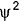) with distance r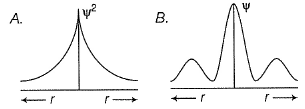Select the correct statement(s).

Solution:

in case of 1s,falls as r increases thus, (A) is for 1s.
In case of 2s,is maximum in the vicinity o f the nucleus, falls to zero giving spherical nodes and then rises to second highest value. Thus, (6) is for2s

QUESTION: 5

Select the correct statement(s).

Solution:

Thus,is d e pendent on r true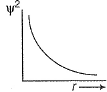(b)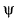and || are dependent on r, θ and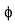: true
(c) Angular wave function is determined by /land m, and not by n: true

QUESTION: 6

Which orbital gives an electron, a greater probability being found close to the nucleus?

Solution:

3s is spherically symmetrical and its electron density is maximum at the nucleus. It decreases with r.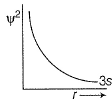QUESTION: 7

Hamiltonian operator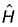is the sum of two energy operators. These are

Solution:

H = T + V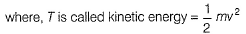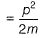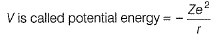QUESTION: 8

Angular nodes in 4s- suborbit is equal to radial nodes in

Solution:

Angular nodes in 4s = 0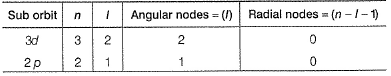QUESTION: 9at any point is proportional to

Solution:

Radial probability density = 4πr2of find in g the electron within the volume of a very thin spherical shell at a distance r from nucleus.

QUESTION: 10

There is formation of in all five nodes (including terminal nodes) in a string of 12 cm. Thus, wavelength of the waves formed is

Solution:

For n nodes in a string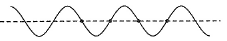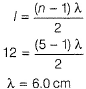QUESTION: 11

For x -axis , wave function () can be written as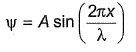Thus, Schrodinger wave equation along x-axis can be written as :

Solution: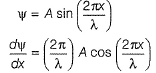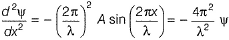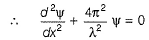QUESTION: 12

The Schrodinger wave equation for H-atom is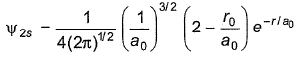where, a0 is Bohr’s radius. If radial node in 2s is at distance r0, then

Solution: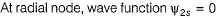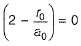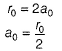*Multiple options can be correct
QUESTION: 13

Direction (Q. Nos. 13-15) This section contains 3 multiple choice questions. Each question has four choices (a), (b), (c) and (d), out of which ONE or  MORE THANT ONE  is correct.

Q.  Which of the following properties can be described by wave function?

Solution:

The wave function describ es properties of the orbital and the electron that occupies the orbital
(a) Type of orbital is described : true
(b) Energy of electron : true
(c) Shape of the orbital: true
(d) Probability: true

*Multiple options can be correct
QUESTION: 14

As compared to 1s electron of H-atom in ground state, which of the following properties appear(s) in the radial probability density of 2s electron of H-atom in first excited state?

Solution:

For2s-electron of H-atom (first excited state)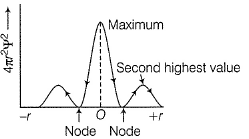For 1s-electron of H-atom in ground state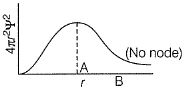At point Ar = a0 r at point Br > a0
(a) True (b) True (c) True (d) True

*Multiple options can be correct
QUESTION: 15

Radial probability density in the occupied orbital of a hydrogen atom in the ground state (1s) is given below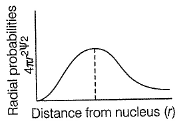Solution:

Radial probability increases as r increase reaches a maximum value when r = a0 (Bohr’s radius) and then falls. When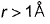radial probability is very small.
Thus, (a) and (c) are true.